# Fractals in Nature: An introduction to IFS and L-System Fractals by Chris Byrd

This presentation gives an introduction to two different types of fractal generation: Iterated Function Systems (IFS) and L-Systems.

## An Introduction to Fractals

### What is a Fractal?

The Presence of fractal geometries occurs throughout nature. A general discription of a fractal could be a geometrical object that visually has the following two properties: It is infinitely detailed and self-similar. Fractals can be seen throughout nature, in plants, in clouds, in mountains just to name a few.

### How are fractals generated?

Most fractals are generating by taking a set of inputs and applying them as inputs to some sort of equation. The output of this equation is then fed into itself again. This feedback is repeated over and over again until the desired number of iterations passes, or until the behavior of the values is determined. Depending on what sort of fractals you are dealing with the criteria for stopping are different.

### Some different types of Fractals

There are various systems for generating different types of fractals. One of the classic examples of a fractal is the Mandlebrot.The Mandlebrot family of fractals is generated by using some variation of the quadratic function Q(z)= z^2 + c where both z and c are complex numbers. Many a fantastic image can be created this way.

The two systems we will discuss here are IFS and L-Systems, these two systems have some interesting properties that allow them to generate some complex images that might occur in nature (clouds, plant growth etc).

## An example of a fractal generated by L-Systems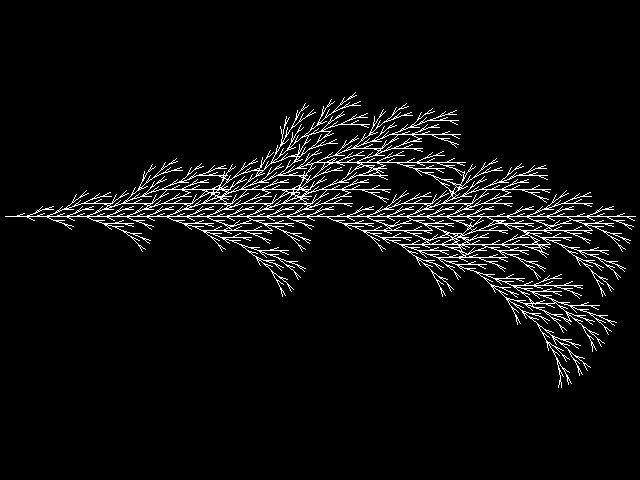## An example of a fractal generated by IFS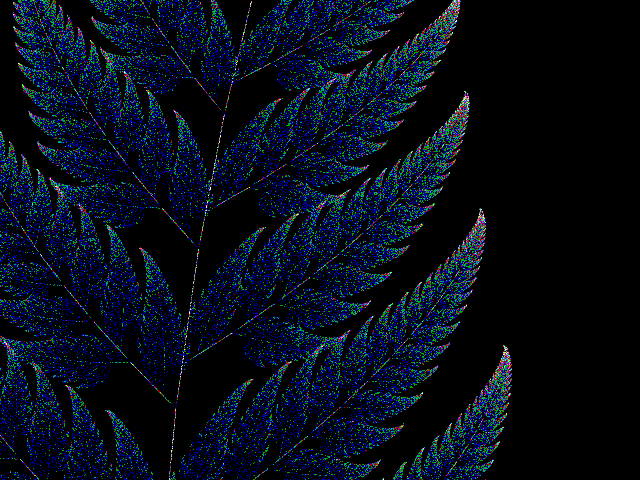## Iterated Function System (IFS)

### The Chaos Game

Imagine you have three arbitrary points on a plane, and a dice with three sides on it. Each side on the die represents one of the above three points. Pick a fourth point anywhere on this plane. Now roll the die. Measure the distance between the fourth point and the stationary point represented by the die, and move your new point to the half-way mark. Now using this new point repeat the process, each time rolling the die and makeing a new point halfway between your current point and the point specified on the die. Do this for many iterations, and what do you get? At first you might guess a bunch of random dots. But in fact the image that you get is shown below. It is what is called the Sierpinski triangle or gasket.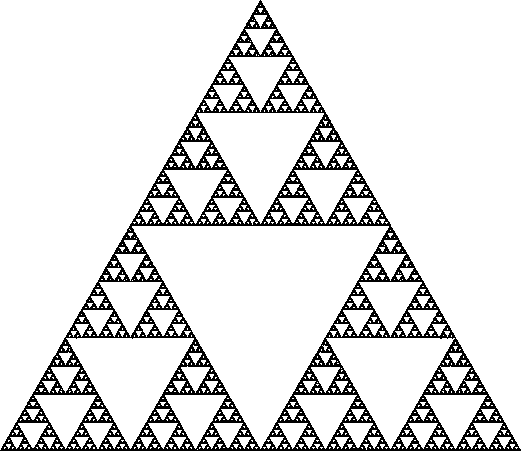This sort of process forms the basis of how IFS works.

### The basics of IFS

An Iterated Function System is comprised of a set of transformations, w1,w2...wn. Each of these transformation can be just about any normal affine transformation. The only restriction is that they must be a contracting transformation. Meaning that if you apply the trandformation it moves two points closer together. The transformations can be written in matrix notation as:

```  | x |   | a   b | | x |   | e |
w |   | = |       | |   | + |   |
| y |   | c   d | | y |   | f |
```

Each of those transformation w1,w2...wn each has a repective value p1,p2...pn that represents the probability that a particular transformation is chosen. The sum of p1,p2...pn must be equal to 1.

Now it is a simple matter to just plug and chug - Pick any initial point as your starting value to begin the feedback loop. Depending on a random number, pick your next transformation, calculate the next value and repeat as long as you want. For each new point you calculate place a pixel on your display at that location. Instead of just using a single pixel, you could instead draw a polygon that has undergone that same transformation.

This process can also very easily extended into three dimensions by using a 3D transformation instead of a 2D one.

You'll probally need at least several thausand iteration to get an idea of what your picture looks like. Even more to get it down to any detail.

### Generating a particular picture with IFS

The Fern IFS, originally found by Michael Barnsley, is a well known and simple IFS that generates the picture of a Black Spleenwort fern.The Fern is created using the following 4 affine transformation:

```
Given the following affine transformation:

| x |   | r*cos(a)   -s*sin(b)  | | x |   | h |
w1 |   | = |                       | |   | + |   |
| y |   | r*sin(a)   s*cos(b)   | | y |   | k |

Translation    Rotation    Scaling
h,k           a,b        r,s

w1      0,0           0,0        0,0.16
w2      0,1.6      -2.5,-2.5  0.85,0.85
w3      0,1.6        49,49     0.3,0.3
w4      0,0.44      120,-50    0.3,0.37

```

### Finding the IFS to fit your image

So far we have looked at some interesting ways to generate some fractal images but what do we do if we have an image of a particular plant, or cload in mind and want to find the IFS to generate an approximation of it?

This is actually not as difficult as it might at first seem. Using the following algorithm (based on the mathematics in the Collage Theorem) one can generate an IFS that can produce your desired image.

The idea is to take the image you are looking to create an IFS for, say a leaf. Take the image of the leaf, and scale it down, rotate and translate it so that this smaller version of the image fits in its entirety within the larger version. This tranformation you just perform becomes the first of your set of transformation, w1. Now again take the original image, scale, rotate and translate it so that fits in a subset of the orinigal that wasn't covered by your previous transform. It is ok if they overlap, however minimizing the overlap is better. Repeat this process till all of the original image is made up of smaller copies of itself. The set of tranformation that you use becomes the set of transforms for your IFS.

## L-Systems

L-Systems are another form of Fractal that have been use to generate imgages that imitate nature. L-systems were created by A. Lindenmayer in 1968 as a method for modeling the growth of living organisms. They wern't encorporated into computer graphics until the mid-80's.

L-systems or string rewriteing systems use a set of productions to generate a representation of an image. An image is determined by three things. The 'axiom' which is the starting symbol or symbols. The set of production rules, and the number of iterations to carry out.

The string that is created is a set of instructions, that specify simple actions that can be used to control a 'turtle' in a similar manner as in LOGO.

```
For example, given the following rules:

F -> F++F-F-
+ -> F-F

And a starting symbol of 'F'.

After one iteration we get 'F++F-F-'.
After two iterations: 'F++F-F-F-FF-FF++F-F--F++F-F--'

etc.

```

Each character specifies an action. F could mean forward one step, a '+' turn right and a '-' turn left. For turns there is a set value for the degree of rotation.

The following table represents some of the various instructions you can give your 'turtle':

```Symbol                  Action
-------------------------------------
F 			Move forward one unit
f			Move forward one units without drawing
+			Turn left by turning angle
-			Turn right by turning angle
|			turn 180 degrees
[			Save the state of the turtle on a stack
]			Pop the state from the stack and set the turtle to it

```

Using this method some fairly one can generate various plant-like creations.

```Using the following rules a bush like plant can be created:

Axiom= ++++F
F= FF-[-F+F+F]+[+F-F-F]
angle=16

```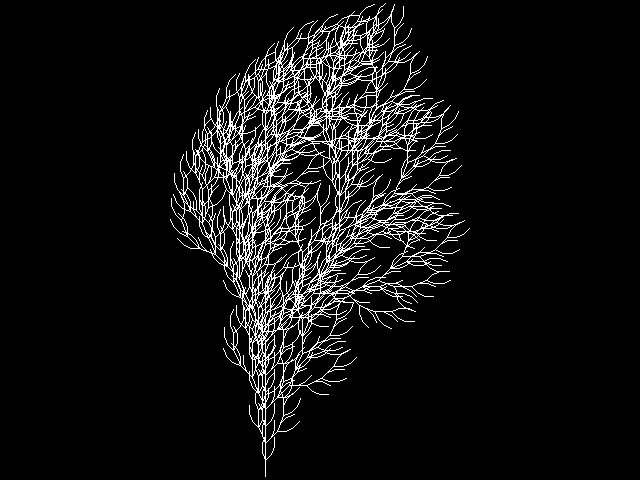L-systems can also be easily extended into 3d. In 2D L-Systems the turtles state is represented by the three values (X,Y,Angle). Hung-Wen Chen developed a 3d L-system generator using the following method. In order to generate a 3D L-system you would start by increasing the turtles state values to (X,Y,Z,Angle1, Angle2, Angle3) The Angles specify the 3D direction the turtle is facing, and X,Y,Z its current location. Angle1 and Angle2 are perpendicular to each other, Angle3 is the normal to the plane that Angle1 and Angle2 form.

Six new sybols were introduced to allow the turtle to turn left or right along any of the three axis. Now one can move the turtle about in 3D.

## A 3D L-System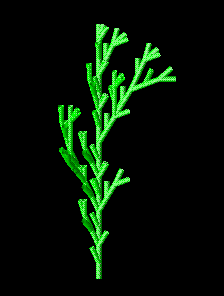References:

 Heinz-Otto Peitgen, Dietmar Saupe, "The Science of Fractal Images."

 Robert L. Devaney, "A first Course in Chaotic Dynamical Systems: theory and experiment"

 An introduction to Fractals

 3D L-systems